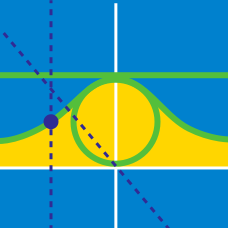Calculus

# Parametric Equations - Derivative

If $x = t^3$ and $y = 3t^5$, where $t$ is any real number, what is the derivative of $y$ with respect to $x$ at $x = 216$?

What is the equation of the tangent line to the curve $x = 14 \sin t, y = 5\ \sin(t + \sin t)$ at $(0,0)$?

If $x = \ln t$ and $y = 3 t^3 + 9 \ln t$ what is $\displaystyle{\frac{d y}{d x}}?$

If $x=t+\frac{1}{t}$ and $y=t^2+\frac{1}{t^2}$, what is the derivative of $y$ with respect to $x$ at $x=21$?

At time $t$, the coordinates of a point $P$ are given by $(4 \cos t, 2 \sin t)$. At time $t=\frac{\pi}{4}$, the magnitudes of velocity and acceleration are $m$ and $n$, respectively. What is $m^2+n^2$?

×